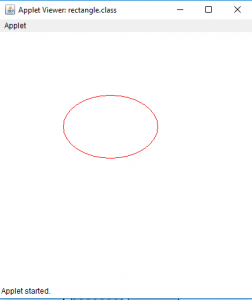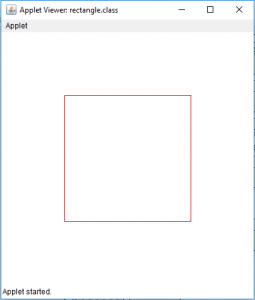# Draw a ellipse and a rectangle in Java Applet

Java applets are application that can be executed in web browsers or applet viewers . We can draw shapes on the Java applet.
In this article we will draw a ellipse on Java applet by two ways . By using the drawOval(int x, int y, int width, int height) or by using mathematical formula (X= A * sin a, Y= B *cos a, where A and B are major and minor axes and a is the angle ) .
Similarly, we will draw a rectangle on Java applet by two ways . By using the drawRect(int x, int y, int width, int height) or by drawing four lines joining the edges .

To draw a ellipse in Java Applet
Examples:
Let us draw a oval with width 150 and height 100

```Input : x and y coordinates 100, 100 respectively
Width and height 150 and 100 respectively
```

Output :To draw a rectangle in Java Applet
Examples:
We will draw a rectangle of height 200 and width 200 and
At a position 100,100 on the applet.

```Input : x and y coordinates 100, 100 respectively
Width and height 200 and 200 respectively.
```

Output :1. Java Program to draw a ellipse using drawOval(int x, int y, int width, int height)

 `// java program to draw a ellipse ` `// using drawOval function. ` `import` `java.awt.*; ` `import` `javax.swing.*; ` ` `  `public` `class` `ellipse ``extends` `JApplet { ` ` `  `    ``public` `void` `init() ` `    ``{ ` `        ``// set size ` `        ``setSize(``400``, ``400``); ` ` `  `        ``repaint(); ` `    ``} ` ` `  `    ``// paint the applet ` `    ``public` `void` `paint(Graphics g) ` `    ``{ ` `        ``// set Color for rectangle ` `        ``g.setColor(Color.red); ` ` `  `        ``// draw a ellipse ` `        ``g.drawOval(``100``, ``100``, ``150``, ``100``); ` `    ``} ` `} `

Output :2. program to draw a ellipse using drawLine function

 `// java program to draw a ellipse ` `// using drawLine function ` `import` `java.awt.*; ` `import` `javax.swing.*; ` ` `  `public` `class` `ellipse ``extends` `JApplet { ` ` `  `    ``public` `void` `init() ` `    ``{ ` `        ``setSize(``300``, ``300``); ` `    ``} ` ` `  `    ``public` `void` `paint(Graphics g) ` `    ``{ ` `        ``// center of the ` `        ``int` `cx, cy; ` ` `  `        ``// center of th ellipse ` `        ``cx = ``150``; ` `        ``cy = ``175``; ` ` `  `        ``// major and minor axis ` `        ``double` `A = ``75``, B = ``50``, px = ``0``, py = ``0``; ` ` `  `        ``// set color ` `        ``g.setColor(Color.red); ` ` `  `        ``// draw the ellipse ` `        ``for` `(``int` `i = ``0``; i <= ``360``; i++) { ` `            ``double` `x, y; ` `            ``x = A * Math.sin(Math.toRadians(i)); ` `            ``y = B * Math.cos(Math.toRadians(i)); ` ` `  `            ``if` `(i != ``0``) { ` `                ``// draw a line joining previous and new point . ` `                ``g.drawLine((``int``)px + cx, (``int``)py + cy, ` `                                ``(``int``)x + cx, (``int``)y + cy); ` `            ``} ` ` `  `            ``// store the previous points ` `            ``px = x; ` `            ``py = y; ` `        ``} ` `    ``} ` `} `

Output :Now we will see how to draw a rectangle in a Java Applet

We can draw rectangle in java applet by two ways.
1. Draw a rectangle using drawRect(int x, int y, int width, int height)

 `// Java Program to  Draw a rectangle ` `// using drawRect(int x, int y, int width, int height) ` `import` `java.awt.*; ` `import` `javax.swing.*; ` ` `  `public` `class` `rectangle ``extends` `JApplet { ` ` `  `    ``public` `void` `init() ` `    ``{ ` `        ``// set size ` `        ``setSize(``400``, ``400``); ` ` `  `        ``repaint(); ` `    ``} ` ` `  `    ``// paint the applet ` `    ``public` `void` `paint(Graphics g) ` `    ``{ ` `        ``// set Color for rectangle ` `        ``g.setColor(Color.red); ` ` `  `        ``// draw a rectangle ` `        ``g.drawRect(``100``, ``100``, ``200``, ``200``); ` `    ``} ` `} `

Output:2. Draw a rectangle using drawLine(int x, int y, int x1, int y1)

 `// Java Program  Draw a rectangle ` `// using drawLine(int x, int y, int x1, int y1) ` `import` `java.awt.*; ` `import` `javax.swing.*; ` ` `  `public` `class` `rectangle ``extends` `JApplet { ` ` `  `    ``public` `void` `init() ` `    ``{ ` `        ``// set size ` `        ``setSize(``400``, ``400``); ` ` `  `        ``repaint(); ` `    ``} ` ` `  `    ``// paint the applet ` `    ``public` `void` `paint(Graphics g) ` `    ``{ ` `        ``// set Color for rectangle ` `        ``g.setColor(Color.red); ` ` `  `        ``// draw a rectangle by drawing four lines ` `        ``g.drawLine(``100``, ``100``, ``100``, ``300``); ` `        ``g.drawLine(``100``, ``300``, ``300``, ``300``); ` `        ``g.drawLine(``300``, ``300``, ``300``, ``100``); ` `        ``g.drawLine(``300``, ``100``, ``100``, ``100``); ` `    ``} ` `} `

Output :Note : the following programs might not run in an online compiler please use an offline IDE.

Attention reader! Don’t stop learning now. Get hold of all the important Java and Collections concepts with the Fundamentals of Java and Java Collections Course at a student-friendly price and become industry ready.

My Personal Notes arrow_drop_upCheck out this Author's contributed articles.

If you like GeeksforGeeks and would like to contribute, you can also write an article using contribute.geeksforgeeks.org or mail your article to contribute@geeksforgeeks.org. See your article appearing on the GeeksforGeeks main page and help other Geeks.

Please Improve this article if you find anything incorrect by clicking on the "Improve Article" button below.

Article Tags :
Practice Tags :

Be the First to upvote.

Please write to us at contribute@geeksforgeeks.org to report any issue with the above content.